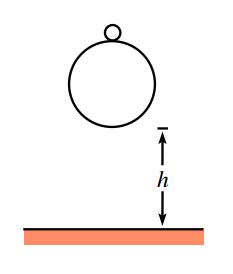A tennis ball with (small) mass $m_2$ sits on top of a basketball with (large) mass $m_1$. The bottom of the basketball is a height $h$ above the ground, and the bottom of the tennis ball is a height $h + d$ above the ground. The balls are dropped. To what height $H$ does the tennis ball bounce?

(Note: Work in the approximation where $m_1$ is much larger than $m_2$, and assume that the balls bounce elastically.)

Question Source - Basketball and tennis ball

Try Part 2 also - Basketball and tennis ball-2!

×